# 卷积神经网络的组件¶

## 卷积层¶$Y^l(m,n) =X^k(m,n)*H^{kl}(m,n) = \sum_{k=0}^{K-1}\sum_{i=0}^{I-1}\sum_{j=0}^{J-1}X^k(m+i,n+j)H^{kl}(i,j)$

• 局部连接 ：假设现在输入图片分辨率是100*100，然后隐藏层神经元有10^5个，如果全连接的话，那么每个隐藏层神经元都连接图像的一个像素点，就有$10^9$个连接，这个参数量是很大的。对于卷积层来讲，如果卷积核大小为$3\times 3$那么每个神经元只需要和原始图像中的一个$3\times 3$的局部区域连接，所以一共只有$9\times 10^5$个连接。可以看到通过局部连接，卷积层的参数量减少了很多。
• 权值共享：在上面的局部连接中，一个有$9\times 10^5$个参数。如果每个神经元对应的参数都相同的话，那么需要训练的参数实际上就只有$9$个了。这个就是$3\times 3$的卷积核。而权值共享的意思就是训练好的一个$3\times 3$卷积核表示了在图像中提取某种特征的能力，例如提取人脸上的眼睛，也就是说卷积核具有了这种能力，无论在图像的哪个地方都可以起作用。这只是一个卷积核的作用，作用肯定是有限的，那么如果我们用100个卷积核来提取特征，那么特征提取能力就会大大增强。同时参数两也不过才$3\times 3\times 100$。所以是非常cheap并且effective的。

## 池化层¶

• 邻域大小受限造成的估计值方差增大。
• 卷积层参数误差造成估计均值的偏移。

• 不变性，更关注是否存在某些特征而不是特征具体的位置。可以看作加了一个很强的先验，让学到的特征要能容忍一些的变化。（实际上这个容忍能力是非常有限的）
• 减少下一层输入大小，减少计算量和参数量。
• 获得定长输出。（文本分类的时候输入是不定长的，可以通过池化获得定长输出）
• 防止过拟合或有可能会带来欠拟合。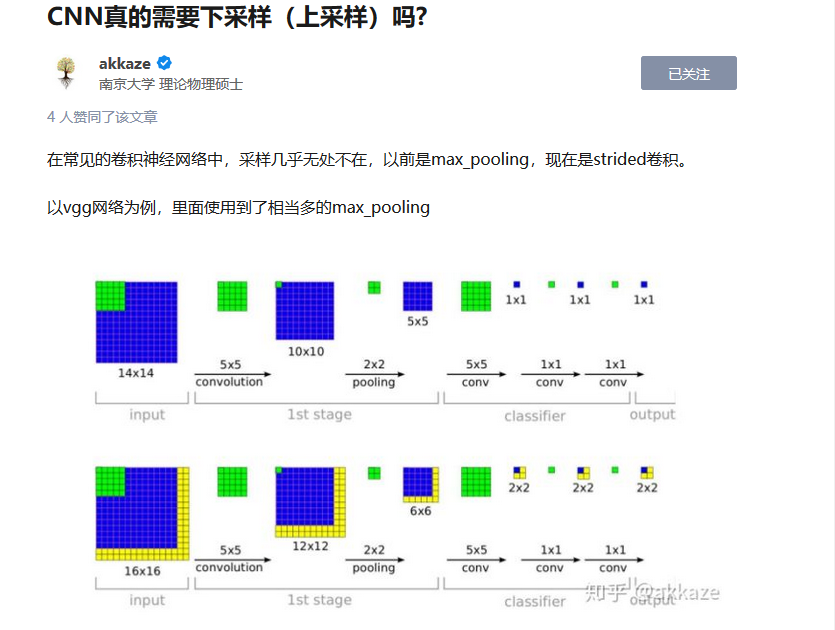# 卷积层是如何在图像中起作用的？¶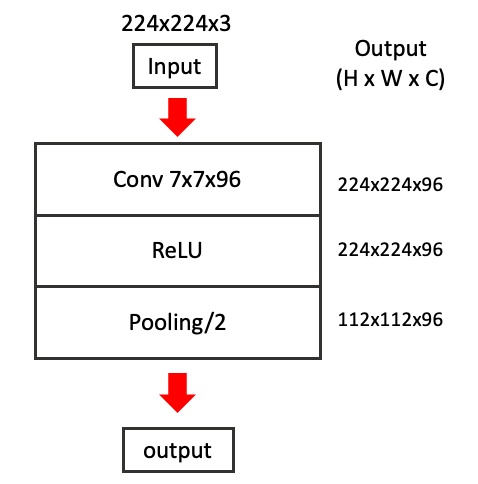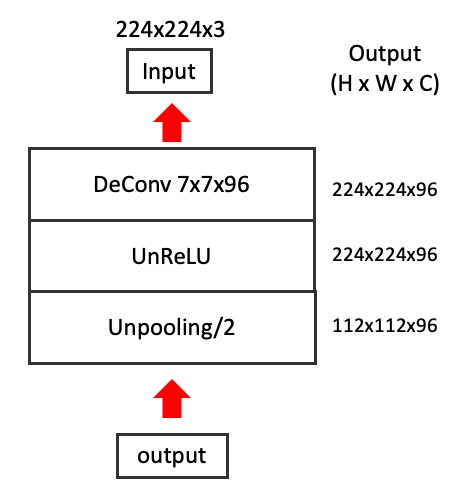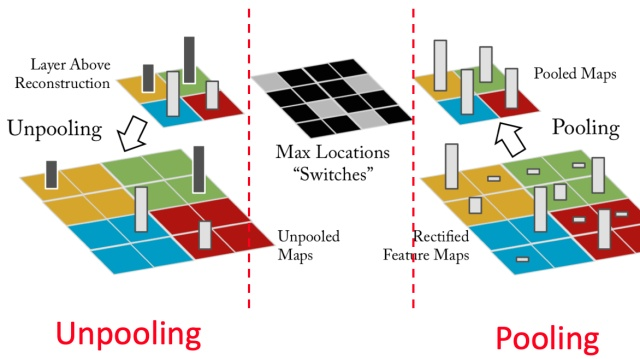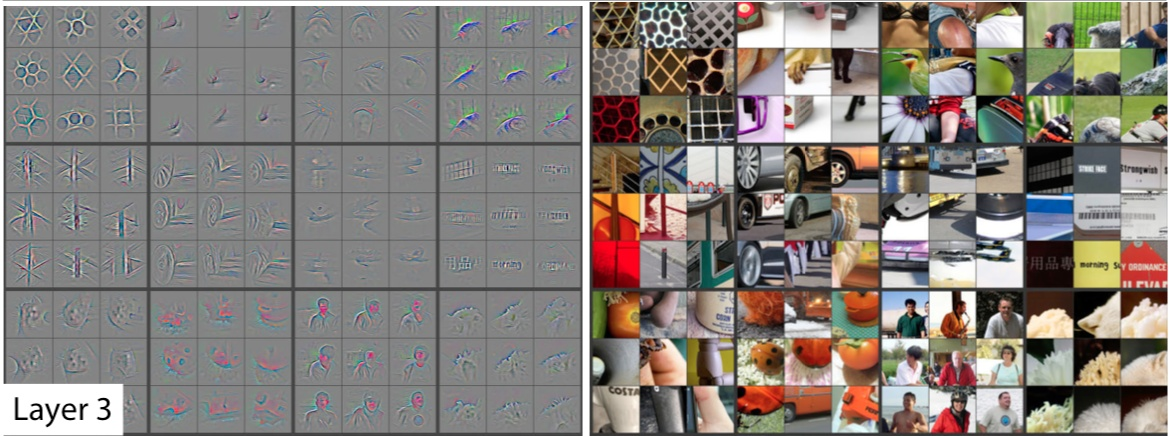# 参考文章¶